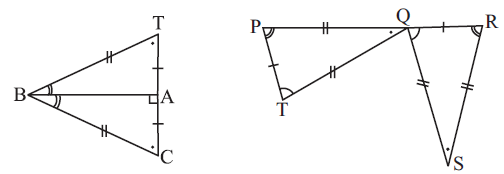# Ex.7.2 Q6 Congruence of Triangles - NCERT Maths Class 7

Go back to  'Ex.7.2'

## Question

Complete the congruence statement:(i) $$ΔBCA ≅ \,\,?$$

(i) $$ΔQRS ≅\,\,?$$

Video Solution
Congruence Of Triangles
Ex 7.2 | Question 6

## Text Solution

What is known?What is the unknown?

Congruence of triangles.

(i) $$ΔBCA ≅ \,\,?$$

(ii)  $$ΔQRS ≅\,\,?$$

From figure, in the triangles $$BTA$$ and $$BCA,$$

$$B$$$$B,$$ $$A$$$$A$$ and $$T$$$$C$$

And in triangle $$QRS$$ and $$TPO,$$

$$P$$$$R,$$ $$T$$$$Q$$ and $$O$$$$S$$

Reasoning:

This question is based on the concept of congruence of triangles, if the corresponding parts of two triangles are equal then the two triangles are congruent to each other. In first triangle, corresponding parts of triangle $$BTA$$ are congruent to the triangle$$BCA$$ and the corresponding parts of triangle $$QRS$$ are congruent to triangle $$TPO.$$ Thus, by applying congruence based on sides of a triangle, two triangles can be proved congruent.

Steps:

In triangle $$BAT$$ and triangle $$BCA,$$ Corresponding parts are congruent.

$$B$$$$B,$$  $$A$$$$A$$ and $$T$$$$C.$$

So, by $$SSS$$ congruence rule, $$ΔBCA ≅ BTA$$

Similarly, in triangle $$QRS$$ and $$TPO,$$ corresponding parts are congruent. So, by $$SSS$$ congruence rule,

$$P$$$$R,$$ $$T ↔ Q$$ and $$O ↔ S$$

$$ΔQRS ≅ TPO$$

Learn from the best math teachers and top your exams

• Live one on one classroom and doubt clearing
• Practice worksheets in and after class for conceptual clarity
• Personalized curriculum to keep up with school